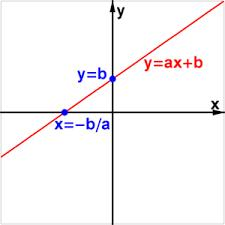# Equation: 6200

Solve the equation:

2y = 4 (0.5 + 3/2) -1

y =  3.5

### Step-by-step explanation:Did you find an error or inaccuracy? Feel free to write us. Thank you!

Tips for related online calculators
Do you have a linear equation or system of equations and looking for its solution? Or do you have a quadratic equation?